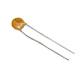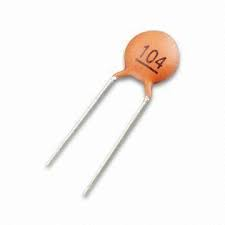# One of the many capacitor numbering schemes

This is a tiny ceramic capacitor.  You can only read the stuff printed on it under high magnificationLet's say that this is the same capacitor, but under high magnification. But what does '104' mean? Is it some kind of model number? Inventory number? Does it mean nothing to anyone but space aliens?Nope. It tells you it's capacitance, in picofrads. The first two digits indicate part of it's capacitance value. The third digit indicates the number of zeros which follow the first two digits.

Thus, it's capacitance is 10, followed by four zeros, i.e., 100,000 picofarads (100,000 pF)

You can also just drop the last three zeros and call it 100 nanofarads (100 nF). Both are the same value; they're just  written differently.

You can find out a bit more here.

And even more, in a straight-to-the-point video, here.